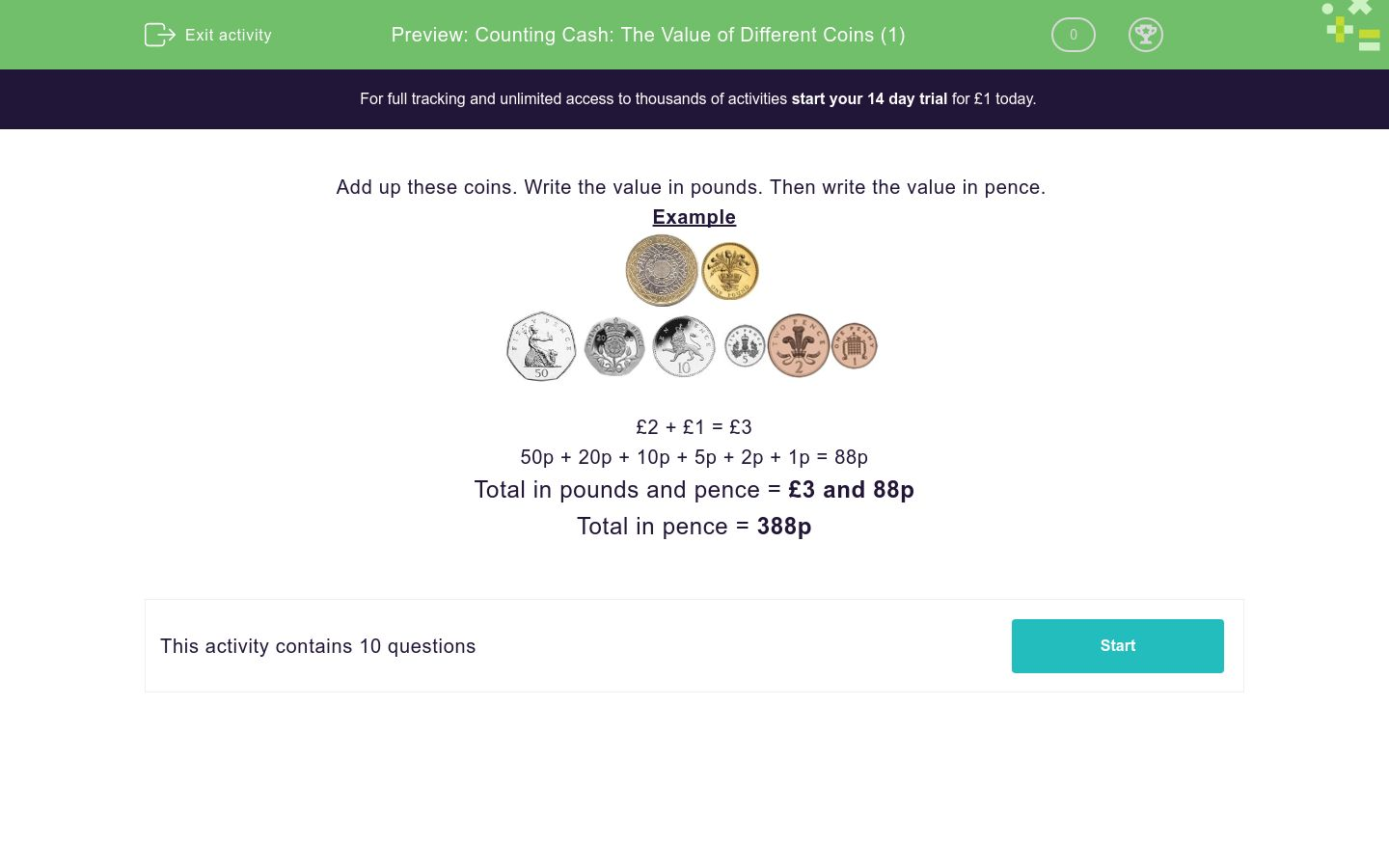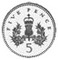# Counting Cash: The Value of Different Coins (1)

In this worksheet, students add together the value of different coins.Key stage:  KS 1

Curriculum topic:   Measurement

Difficulty level:### QUESTION 1 of 10

Add up these coins. Write the value in pounds. Then write the value in pence.

Example£2 + £1 = £3

50p + 20p + 10p + 5p + 2p + 1p = 88p

Total in pounds and pence = £3 and 88p

Total in pence = 388p

Find the total of these coins. TWO boxes are correct. Click them.£4 and 50p

350p

£3 and 50p

Find the total of these coins. TWO boxes are correct.£3

£4

3

300p

Find the total of these coins. TWO boxes are correct. Click them.£3 and 75p

£3 and 70p

370p

Find the total of these coins. TWO boxes are correct. Click them.£3 and 80p

£3 and 90p

380p

Find the total of these coins. TWO boxes are correct. Click them.470

470p

£4 and 70p

Find the total of these coins. TWO boxes are correct. Click them.£3 and 1p

£2 and 1p

210p

201p

Find the total of these coins. TWO boxes are correct. Click them.£2 and 10p

201p

210p

£4 and 10p

Find the total of these coins. TWO boxes are correct. Click them.£5 and 50p

£3 and 50p

350p

350

Find the total of these coins. TWO boxes are correct. Click them.£5 and 5p

£3 and 5p

350p

305p

Find the total of these coins. TWO boxes are correct. Click them.268p

£1 and 68p

618p

168p

• Question 1

Find the total of these coins. TWO boxes are correct. Click them.350p
£3 and 50p
EDDIE SAYS
Either £3 and 50p or 350p works for this one.
• Question 2

Find the total of these coins. TWO boxes are correct.£3
300p
EDDIE SAYS
£3 or 300p is correct for this question.
• Question 3

Find the total of these coins. TWO boxes are correct. Click them.£3 and 70p
370p
EDDIE SAYS
We are looking for either £3 and 70p or 370p.
• Question 4

Find the total of these coins. TWO boxes are correct. Click them.£3 and 80p
380p
EDDIE SAYS
Here we can have either £3 and 80p or 380p.
• Question 5

Find the total of these coins. TWO boxes are correct. Click them.470p
£4 and 70p
EDDIE SAYS
Remember, we do NOT write 470. We write 470p or £4 and 70p.
• Question 6

Find the total of these coins. TWO boxes are correct. Click them.£2 and 1p
201p
EDDIE SAYS
Look hard.
There are 2 pounds.
There is 1 penny.
So we have £2 and 1p or 201p.
• Question 7

Find the total of these coins. TWO boxes are correct. Click them.£2 and 10p
210p
EDDIE SAYS
Look hard.
We have £2.00 + 10p
That makes £2 and 10p
or 210p.
• Question 8

Find the total of these coins. TWO boxes are correct. Click them.£3 and 50p
350p
EDDIE SAYS
Remember, we write 350p or £3 and 50p
• Question 9

Find the total of these coins. TWO boxes are correct. Click them.£3 and 5p
305p
EDDIE SAYS
Look hard.
The final coin is 5p.
We can either write £3 and 5p or 305p.
• Question 10

Find the total of these coins. TWO boxes are correct. Click them.£1 and 68p
168p
EDDIE SAYS
Remember:
we write £1 and 68p
or we write 168p.
---- OR ----

Sign up for a £1 trial so you can track and measure your child's progress on this activity.

### What is EdPlace?

We're your National Curriculum aligned online education content provider helping each child succeed in English, maths and science from year 1 to GCSE. With an EdPlace account you’ll be able to track and measure progress, helping each child achieve their best. We build confidence and attainment by personalising each child’s learning at a level that suits them.

Get started